# Precalculus : Trigonometric Functions

## Example Questions

1 3 Next →

### Example Question #16 : Find The Value Of Any Of The Six Trigonometric Functions

Find the value of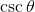.Possible Answers: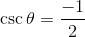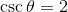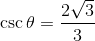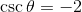Correct answer:Explanation:

Since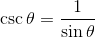we begin by finding the value of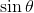.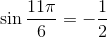.

Then,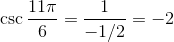### Example Question #17 : Find The Value Of Any Of The Six Trigonometric Functions

Determine the value of: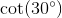Possible Answers: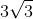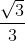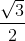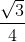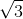Correct answer:Explanation:

To determine the value of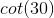, simplify cotangent into sine and cosine.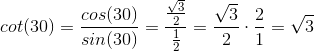### Example Question #18 : Find The Value Of Any Of The Six Trigonometric Functions

Find the value of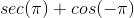, if possible.

Possible Answers: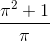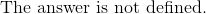Correct answer:Explanation:

In order to solve, split up the expression into 2 parts.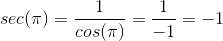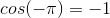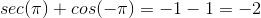### Example Question #19 : Find The Value Of Any Of The Six Trigonometric Functions

Compute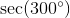, if possible.

Possible Answers: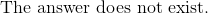Correct answer:Explanation:

Rewrite the expression in terms of cosine.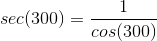Evaluate the value of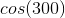, which is in the fourth quadrant.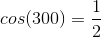Substitute it back to the simplified expression of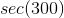.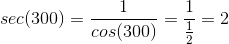### Example Question #20 : Find The Value Of Any Of The Six Trigonometric Functions

Determine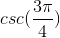Possible Answers: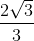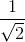Correct answer:Explanation:

Remember that: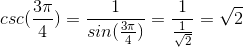### Example Question #21 : Find The Value Of Any Of The Six Trigonometric Functions

What is the value of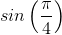?

Possible Answers: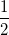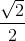Correct answer:Explanation:

The sine of an angle corresponds to the y-component of the triangle in the unit circle.  The angle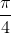is a special angle.  In the unit circle, the hypotenuse is the radius of the unit circle, which is 1.  Since the angle is, the triangle is an isosceles right triangle, or a 45-45-90.

Use the Pythagorean Theorem to solve for the leg. Both legs will be equal to each other.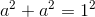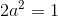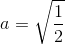Rationalize the denominator.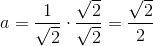Therefore,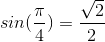.

1 3 Next →

### All Precalculus Resources